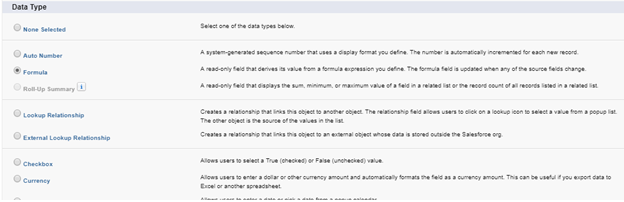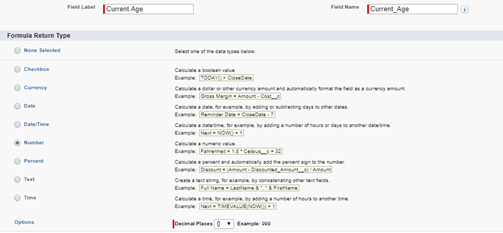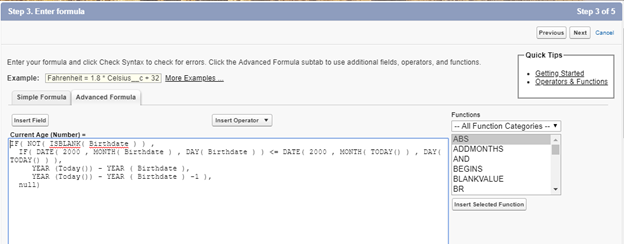1 Comment

To find the age of items such as the age of person, age of an account, age of the client relationship, etc., a custom formula field can be created to calculate the time difference between two dates.

The formula field should be placed on the object it is doing the calculation from.  For example if you want the how long an account has been active, the formula field would be placed on the Account object.  To calculate the age of a Contact based on their birth date, the formula field would be placed on the Contact object.

To create a formula field to calculate age based on a Contact birth date:

• Go to Setup -> Customize -> Contact -> Fields
• In Lighting go to Setup -> Object Manager -> Contact -> Fields & Relationships
• Click New to create a custom field.
• Select Formula from the Data Type List and click Next.• Enter a field label (e.g., Current Age).
• Choose Number for the Return Type with 0 decimal places.• Paste the following formula into the Formula box:
```IF( NOT( ISBLANK( Birthdate ) ) ,
IF( DATE( 2000 , MONTH( Birthdate ) , DAY( Birthdate ) ) <= DATE( 2000 , MONTH( TODAY() ) , DAY( TODAY() ) ),
YEAR (Today()) - YEAR ( Birthdate ),
YEAR (Today()) - YEAR ( Birthdate ) -1 ),
null)```

Translation:  If the Birthdate field is not blank then check to see if the Birthdate month and day are less than or equal to today.  If the Birthdate is less than or equal to today, then subtract the year of Today’s date from the year of the Birthdate.  If the Birthdate is greater than today, then subtract Today’s year from the year of the Birthdate minus 1.If you are using a different date field, replace “Birthdate” with the desired object’s name.

• Click the Check Syntax button at the bottom of the Formula box. If no syntax errors, click Next.
• Choose what user Profiles with be able to see the new field and to edit the field. Keep Read Only checked as the formula is driving the data.
• Click Next
• Choose what Page Layouts the new field will be displayed on. You can go into Page Layouts later to reposition the field on the page.
• Click Save. Go to the Contact page and verify the Current Age field is populating correctly.

Enjoy!

## — One Comment —

1.Ken Tallman says:

Thanks Becci. Handy formula.